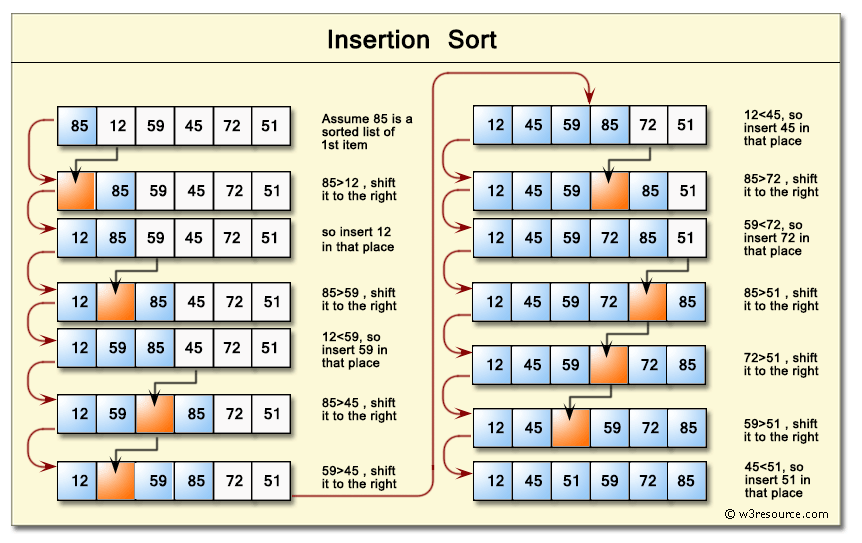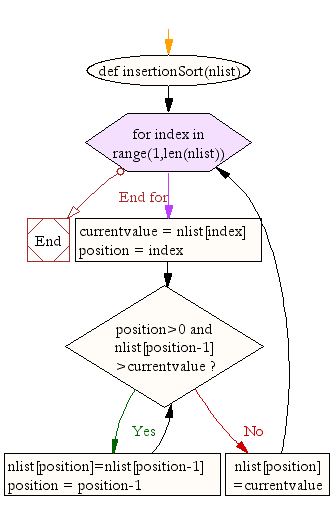﻿ Python: Insertion sort - w3resource# Python: Insertion sort

## Python Search and Sorting : Exercise-6 with Solution

Write a Python program to sort a list of elements using the insertion sort algorithm.
Note: According to Wikipedia "Insertion sort is a simple sorting algorithm that builds the final sorted array (or list) one item at a time. It is much less efficient on large lists than more advanced algorithms such as quicksort, heapsort, or merge sort."

Pictorial Presentation: Insertion SortSample Solution:-

Python Code:

``````def insertionSort(nlist):
for index in range(1,len(nlist)):

currentvalue = nlist[index]
position = index

while position>0 and nlist[position-1]>currentvalue:
nlist[position]=nlist[position-1]
position = position-1

nlist[position]=currentvalue

nlist = [14,46,43,27,57,41,45,21,70]
insertionSort(nlist)
print(nlist)
``````

Sample Output:

```[14, 21, 27, 41, 43, 45, 46, 57, 70]
```

Flowchart :## Visualize Python code execution:

The following tool visualize what the computer is doing step-by-step as it executes the said program:

Python Code Editor :

What is the difficulty level of this exercise?

Test your Programming skills with w3resource's quiz.

﻿

## Python: Tips of the Day

The Zip() Function:

```>>> students = ('John', 'Mary', 'Mike')
>>> ages = (15, 17, 16)
>>> scores = (90, 88, 82, 17, 14)
>>> for student, age, score in zip(students, ages, scores):
...     print(f'{student}, age: {age}, score: {score}')
...
John, age: 15, score: 90
Mary, age: 17, score: 88
Mike, age: 16, score: 82
>>> zipped = zip(students, ages, scores)
>>> a, b, c = zip(*zipped)
>>> print(b)
(15, 17, 16)
```